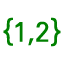# Gauss-Jordan Elimination Toolkit

## Enter equation coefficients into an appropriate box and use the corresponding button to generate the augmented matrix. Then use row operations on the toolbar to solve the system. More help is below.

More help hints: Enter matrix elements in the spreadsheet and generate the desired matrix by using the color-coded buttons. If your matrix has dimensions different from the options given, simply type the elements and use the List/Matrix toolto generate your matrix. Row Operations In general, you first need to specify the row(s), to which you want to apply the row operation and then a constant (if any). More specifically:
• Multiplication by a non-zero constant: Enter the row number and then the constant. For example, if you want to replace R2 with 5R2, you will enter 2 and then 5 when asked to do so.
• Swapping two rows: Enter the row numbers (in any order). For example to swap R1 R3, you will enter 1 and then 3 when asked.
• Adding a multiple of a row to another row: Enter the row you want to multiply, then the row you want to add to (replace) and finally enter the constant. For example, to do 3R1 + R2 R2, you will enter 1, then 2 and then 3.